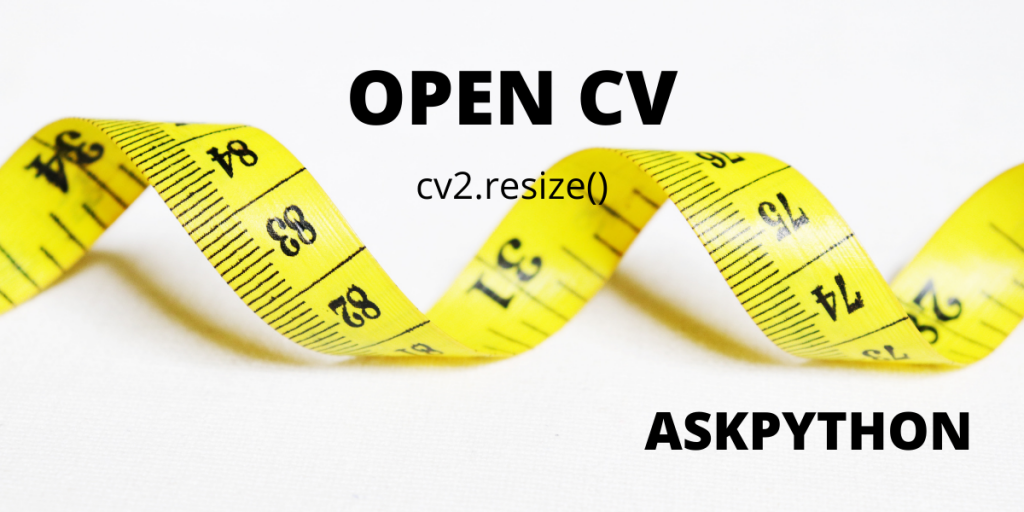# CV2 Resize – A Complete Guide for BeginnersIn this article, we will be learning about the OpenCV package, and its cv2 resize function. We will be looking at a few code examples as well to get a better understanding of how to use this function in practical scenarios.

## Open CV – cv2 resize()

This image processing computer library was built by intel to address real-time vision issues in computers. The cv2 resize() function is specifically used to resize images using different interpolation techniques. Let’s understand how.

## Simple Resizing

```import cv2
bigger = cv2.resize(image, (2400, 1200))
cv2.imshow("Altered Image", bigger)
cv2.waitKey(0)
cv2.destroyAllWindows()
```

The above code demonstrates a simple resizing technique. Here we did not use any interpolation technique or scaling factors but we got the desired output.

We can add scaling factors to our syntax as well. Scaling factors scales the image along their axes, without adding much difference to the final output. The syntax with scaling factors is written something like:

```scaled = cv2.resize(image, (1200, 600), fx = 0.1, fy = 0.1
,interpolation = cv2.INTER_CUBIC)
```

## Resizing by changing the aspect ratio

Changing the aspect ratio of the image can give us a shrank or an enlarged image. In this example, we will be looking at how that can be done.

We will be looking at 3 blocks of code, which involves – package import & image loading, the logic used behind scaling the loaded image, and lastly, resizing the image using interpolation.

```import cv2

```

The code above imports the OpenCV library for Python then loads the image in the variable ‘pic’. You might have noticed, we used ‘cv2.IMREAD_UNCHANGED’, its basic function is to load the image using its alpha channel, which means the original resolution of the pic gets preserved.

### Algorithm for changing the aspect ratio

```pd_change = 60  # The percent change from the main aspect ratio
new_resolution = pd_change/100
pic_width = int(pic.shape * new_resolution)
pic_height = int(pic.shape * new_resolution)
new_dimension = (pic_width, pic_height)
```

Let’s understand the above code line by line:

• ‘pd_change’ variable saves the required percent chage of the original aspect ratio.
• ‘new_resolution’ variable converts that percentage into decimal and stores it.
• Variables ‘pic_width’ and ‘pic_height’ saves new height and width using that decimal value. Syntax ‘pic.shape’ is used to fetch the aspect ratio of the original picture. The  indicates to height and  indicates width. (The  is used for channels which is outside the scope of the learnings involved in this article)
• Variable ‘new_dimension’ is used to store the new resolution.

### Resizing Image

```altered_size = cv2.resize(pic, new_dimension, interpolation=cv2.INTER_AREA)

cv2.imshow("Altered Image", altered_size)
```

Variable ‘altered_size’ resizes the image using cv2.resize() function, the interpolation method used here is ‘cv2.INTER_AREA’, which is basically used to shrink images. So, at last, we got our image scaled perfectly to the percent size we wanted.

Let’s look at the complete code to get the whole picture.

```import cv2

print('Resolution of the original pic : ', pic.shape)

percent_dim_change = 60  # The percent change from the main size
pic_width = int(pic.shape * percent_dim_change / 100)
pic_height = int(pic.shape * percent_dim_change / 100)
dim = (pic_width, pic_height)

# resizing image
altered_size = cv2.resize(pic, dim, interpolation=cv2.INTER_AREA)

print('Changed Picture Dimensions : ', altered_size.shape)

cv2.imshow("Altered Image", altered_size)
cv2.waitKey(0)
cv2.destroyAllWindows()
```

### Resizing by using custom values

We can resize images with specific width and height values, irrespective of their original dimensions. Or, we can change a single parameter, i.e, height, while keeping the other parameter, i.e, width, preserved. Let’s look at the code to understand how it’s done.

```import cv2

print('Resolution of the original pic : ', pic.shape)

pic_width = pic.shape  # keeping intact the original width
pic_height = 800
new_dimension = (pic_width, pic_height)

# resize image
altered_size = cv2.resize(pic, new_dimension, interpolation=cv2.INTER_CUBIC)

print('Changed Picture Dimensions : ', altered_size.shape)

cv2.imshow("Resized image", altered_size)
cv2.waitKey(0)
cv2.destroyAllWindows()
```

Here, we used a different interpolation method, ‘INTER_CUBIC’, which interpolates the 2X2 neighboring pixels. Changing the interpolation method does not make much of a difference and the final result is nearly the same in this example (As a practice exercise, You can try out this example in your machine by changing the interpolation methods, and observing the change in the final result). Nonetheless, In the final output, we get a new image with an altered height.

Note: In the above code, we changed the height of the picture, and also kept our width intact. In case, we want to change the width while keeping the height intact, we will be using:

```pic_width =  480
pic_height = pic.shape     # keeping intact the original height
new_dimension = (pic_width, pic_height)
```